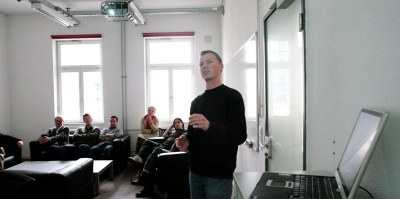You are here: Home Tools

# Tools

We investigate tools that automatically analyze and verify properties of systems (at all levels of the software development process, i.e.,whether the system is only a requirement specification, already a design model or even a piece of program code). The tools are semantics-based. This means that they manipulate their input on an abstract level, in the same way that we abstract a mathematical object by its meaning in a mathematical theory (e.g., a fraction by a rational number). The tools delegate part of their work to dedicated modules (constraint solvers, decision procedures, theorem provers) that account for the mathematical theories for data and control constructs.A part of the difficulty (and attractiveness) of our research activity is that we must (and can) first define the mathematical theory for the input to our tools, and define the analysis and verification problem as an algorithmic problem (which is when the fun part starts).